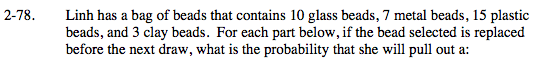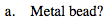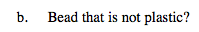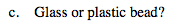### Home > MC2 > Chapter 2 > Lesson 2.2.4 > Problem2-78

2-78.Refer to problem 2-50 for more help.Determine the number of beads in the bag.
Total number of beads = 35

Divide the number of metal beads by the total number

$\frac{7}{35}$

$\frac{7}{35}=\frac{1}{5}$Use the same method as part (a).

$\frac{20}{35}=\frac{4}{7}$Use the same method as part (a).

Use the eTool below to test the probability of each part.
Click the link at right for the full version of the eTool: MC2 2-78 HW eTool